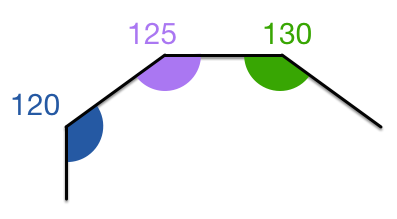# Polygon with increasing anglesThe interior angles of a convex polygon are in arithmetic progression. The smallest angle is $120^\circ$ and the common difference is $5^\circ$.

Find the number of sides of the polygon.

×